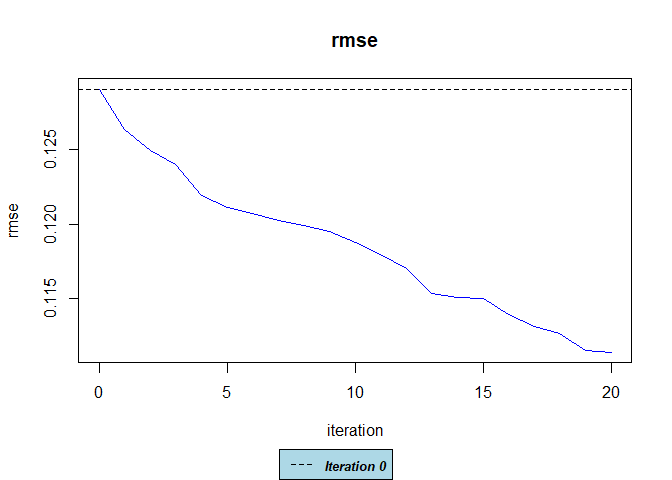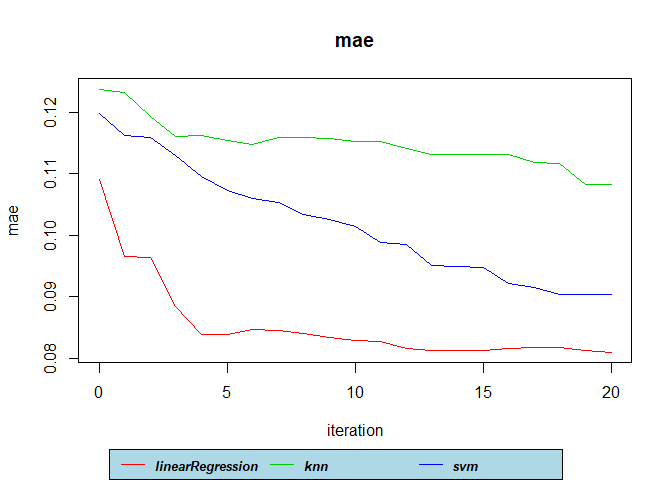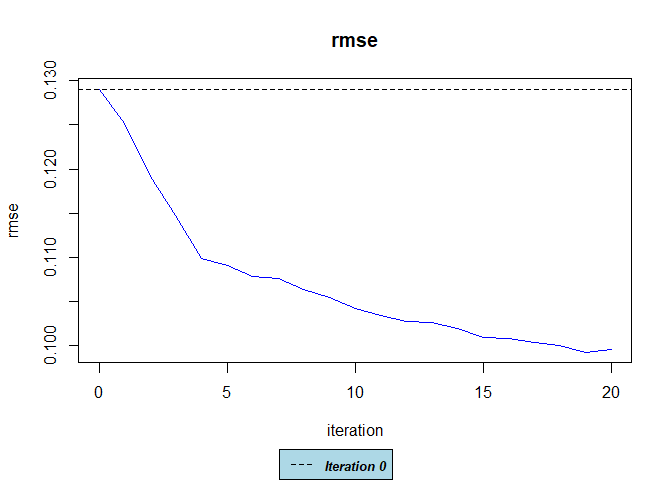Introduction

This package implements the self-learning and co-training by committee semi-supervised regression algorithms from a set of n base regressor(s) specified by the user. When only one model is present in the list of regressors, self-learning is performed. The co-training by committee implementation is based on Hady et al. (2009). It consists of a set of n base models (the committee), each, initially trained with independent bootstrap samples from the labeled training set L. The Out-of-Bag (OOB) elements are used for validation. The training set for each base model b is augmented by selecting the most relevant elements from the unlabeled data set U. To determine the most relevant elements for each base model b, the other models (excluding b) label a set of data pool.size points sampled from U by taking the average of their predictions. For each newly labeled data point, the base model b is trained with its current labeled training data plus the new data point and the error on its OOB validation data is computed. The top gr points that reduce the error the most are kept and used to augment the labeled training set of b and removed from U.

When the regressors list contains a single model, self-learning is performed. That is, the base model labels its own data points as opposed to co-training by committee in which the data points for a given model are labeled by the other models.

In the original paper, Hady et al. (2009) use the same type of regressor for the base models but with different parameters to introduce diversity. The ssr function allows the user to specify any type of regressors as the base models. The regressors can be models from the caret package, other packages, or custom functions. Models from other packages or custom functions need to comply with certain structure. First, the model’s function used for training must have a formula as its first parameter and a parameter named data that accepts a data frame as the training set. Secondly, the predict() function must have the trained model as its first parameter and a data frame as a second parameter. Most of the models from other libraries follow this pattern. If they do not follow this pattern, you can still use them by writing a wrapper function (See section ‘Custom Functions’).

This document explains the following topics:

• Fit semi-supervised regression models with the ssr function.
• Plotting the results.
• Defining the regressors and regressors.params lists.
• Specify regressors from custom functions.
• Training an Oracle model.

Fitting your first model with ssr

Throughout this document we will be using the Friedman #1 dataset. An instance of this dataset is already included in the ssr package. The dataset has 10 input variables (X1..X10) and 1 response variable (Ytrue), all numeric. For more information about the dataset type ?friedman1.

library(ssr)

dataset <- friedman1 # Load friedman1 dataset.

#>          X1        X2         X3         X4        X5         X6        X7
#> 1 0.1134795 0.8399474 0.11267556 0.96430749 0.1644563 0.08368120 0.3505353
#> 2 0.6226043 0.4880453 0.19107638 0.20620675 0.7157168 0.17017763 0.3233741
#> 3 0.6095661 0.1090480 0.61859262 0.08544048 0.4603640 0.70467854 0.6984391
#> 4 0.6236855 0.3512679 0.59912416 0.21548785 0.6389154 0.65350053 0.2480377
#> 5 0.8614685 0.7629973 0.06036928 0.23914582 0.4559488 0.09086521 0.6226571
#> 6 0.6406343 0.3897594 0.69961305 0.19658927 0.9485355 0.71688258 0.5748716
#>           X8        X9        X10     Ytrue
#> 1 0.02669855 0.1675178 0.08034975 0.5417472
#> 2 0.67944169 0.4657235 0.03162659 0.5153556
#> 3 0.18961889 0.2078145 0.18109117 0.1321456
#> 4 0.91980858 0.7714593 0.08252948 0.3722591
#> 5 0.52383235 0.4238548 0.94166606 0.5780382
#> 6 0.69551194 0.8048951 0.99492079 0.4728131

set.seed(1234)

# Split the dataset into 70% for training and 30% for testing.
split1 <- split_train_test(dataset, pctTrain = 70)

# Choose 5% of the train set as the labeled set L and the remaining will be the unlabeled set U.
split2 <- split_train_test(split1$trainset, pctTrain = 5) L <- split2$trainset # This is the labeled dataset.

U <- split2$testset[, -11] # Remove the labels since this is the unlabeled dataset. testset <- split1$testset # This is the test set.

Now lets define a co-training by committee model with a linear model, a KNN and a SVM as base regressors. Regressors are specified as a list with strings and/or functions. In this case, the first regressor is the linear model lm, the second model is a KNN, and the third one is a support vector machine from the e1071 package. In this case, we are using knnreg from the caret package but this could be from another package.

# Define list of regressors.
regressors <- list(linearRegression=lm, knn=caret::knnreg, svm=e1071::svm)

# Fit the model.
model <- ssr("Ytrue ~ .", L, U, regressors = regressors, testdata = testset)
#>  "Initial RMSE on testdata: 0.1290"
#>  "Iteration 1 (testdata) RMSE: 0.1263 Improvement: 2.11%"
#>  "Iteration 2 (testdata) RMSE: 0.1250 Improvement: 3.17%"
#>  "Iteration 3 (testdata) RMSE: 0.1240 Improvement: 3.91%"
#>  "Iteration 4 (testdata) RMSE: 0.1219 Improvement: 5.51%"
#>  "Iteration 5 (testdata) RMSE: 0.1211 Improvement: 6.18%"
#>  "Iteration 6 (testdata) RMSE: 0.1207 Improvement: 6.49%"
#>  "Iteration 7 (testdata) RMSE: 0.1202 Improvement: 6.82%"
#>  "Iteration 8 (testdata) RMSE: 0.1199 Improvement: 7.07%"
#>  "Iteration 9 (testdata) RMSE: 0.1195 Improvement: 7.42%"
#>  "Iteration 10 (testdata) RMSE: 0.1188 Improvement: 7.95%"
#>  "Iteration 11 (testdata) RMSE: 0.1179 Improvement: 8.60%"
#>  "Iteration 12 (testdata) RMSE: 0.1170 Improvement: 9.30%"
#>  "Iteration 13 (testdata) RMSE: 0.1153 Improvement: 10.63%"
#>  "Iteration 14 (testdata) RMSE: 0.1151 Improvement: 10.84%"
#>  "Iteration 15 (testdata) RMSE: 0.1150 Improvement: 10.89%"
#>  "Iteration 16 (testdata) RMSE: 0.1139 Improvement: 11.73%"
#>  "Iteration 17 (testdata) RMSE: 0.1131 Improvement: 12.33%"
#>  "Iteration 18 (testdata) RMSE: 0.1126 Improvement: 12.71%"
#>  "Iteration 19 (testdata) RMSE: 0.1115 Improvement: 13.57%"
#>  "Iteration 20 (testdata) RMSE: 0.1114 Improvement: 13.69%"

Regressors can also be specified by strings from the caret package:

regressors <- list("lm", "rvmLinear")

or combinations between strings and functions:

regressors <- list("lm", knn=caret::knnreg)

For a list of available regressor models that can be passed as strings from the caret package please see here. For better performance in time, it is recommended to pass functions directly rather than using ‘caret’ strings since ‘caret’ does additional preprocessing when training models and this increases training times significantly.

NOTE: If a regressor is specified as a function (knnreg in the above example), it has to be named. In this case, it was named knn. For regressors specified as strings, names are optional. In the above example, “lm” does not have a name. This is to ensure that the name of the regressor is plotted.

ANOTHER NOTE: When specifying a regressor as a function, that function must accept as its first parameter a formula and another parameter named data that takes a data frame. The parameter data can be at any position of the original function but formula must be the first one. Most functions in other packages follow this pattern. If you want to use a function on a package that does not follow this pattern, you can write a custom wrapper function (See section ‘Custom Functions’). Additionally, the functions predict() method must accept a fitted model as its first argument and a data frame as the second argument.

By default, plotmetrics = FALSE so no diagnostic plots are shown during training. To generate plots during training just set it to TRUE. Since the verbose parameter is TRUE by default, performance information is printed to the console including the initial Root Mean Squared Error (RMSE) and the RMSE during each iteration. The performance information is computed on the testdata, if provided. The initial RMSE is computed when the model is trained just on the labeled data L before using any data from the unlabeled set U. The improvement with respect to the initial RMSE is also shown. The improvement is computed as:

$improvement = \frac{RMSE_0 - RMSE_i}{RMSE_0}$

where $$RMSE_0$$ is the initial RMSE and $$RMSE_i$$ is the RMSE of the current iteration.

You can plot the performance across iterations with the plot() function and get the predictions on new data with the predict() function.

# Plot RMSE.
plot(model)# Get the predictions on the testset.
predictions <- predict(model, testset)

# Calculate RMSE on the test set.
rmse.result <- sqrt(mean((predictions - testset$Ytrue)^2)) rmse.result #>  0.1113865 You can also inspect other performance metrics by specifying the metric parameter to one of: “rmse”, “mae” or “cor”. You can also plot the results of the individual regressors by setting ptype = 2. plot(model, metric = "mae", ptype = 2)Specifying regressors’ parameters with regressors.params You can specify individual parameters (such as k for knn) for each regressor via theregressors.params parameter. This parameter accepts a list of lists. Currently, it is not possible to specify parameters for caret models defined as strings but just for the ones specified as functions. If you do not want to specify parameters for a regressor use NULL. # Prepare data. dataset <- friedman1 set.seed(1234) split1 <- split_train_test(dataset, pctTrain = 70) split2 <- split_train_test(split1$trainset, pctTrain = 5)
L <- split2$trainset U <- split2$testset[, -11]
testset <- split1$testset # Define list of regressors. regressors <- list(linearRegression=lm, knn=caret::knnreg) # Specify their parameters. k = 7 for knnreg in this case. regressors.params <- list(NULL, list(k=7)) model2 <- ssr("Ytrue ~ .", L, U, regressors = regressors, regressors.params = regressors.params, testdata = testset) plot(model2) Training an Oracle model Sometimes it is useful to compare your model against an ‘Oracle’. In this context, an Oracle is a model that knows the true values of the unlabeled dataset U. This information is used when searching for the best candidates to augment the labeled set and once the best candidates are found, their true labels are used to train the models. This can be used to have an idea of the expected upper bound performance of the model. This option should be used with caution and not to be used to train a final model but just for comparison purposes. To train an Oracle model, just pass the true labels to the U.y parameter. When using this parameter, a warning will be printed. # Prepare the data dataset <- friedman1 set.seed(1234) split1 <- split_train_test(dataset, pctTrain = 70) split2 <- split_train_test(split1$trainset, pctTrain = 5)
L <- split2$trainset U <- split2$testset[, -11]
testset <- split1$testset # Get the true labels for the unlabeled set. U.y <- split2$testset[, 11]

# Define list of regressors.
regressors <- list(linearRegression=lm, knn=caret::knnreg, svm=e1071::svm)

# Fit the model.
model4 <- ssr("Ytrue ~ .", L, U,
regressors = regressors,
testdata = testset,
U.y = U.y)
#> Warning in ssr("Ytrue ~ .", L, U, regressors = regressors, testdata = testset, : U.y was provided. Be cautious when providing this parameter since this will assume
#>             that the labels from U are known. This is intended to be used to estimate a performance upper bound.
#>  "Initial RMSE on testdata: 0.1290"
#>  "Iteration 1 (testdata) RMSE: 0.1251 Improvement: 3.02%"
#>  "Iteration 2 (testdata) RMSE: 0.1192 Improvement: 7.66%"
#>  "Iteration 3 (testdata) RMSE: 0.1147 Improvement: 11.11%"
#>  "Iteration 4 (testdata) RMSE: 0.1099 Improvement: 14.84%"
#>  "Iteration 5 (testdata) RMSE: 0.1091 Improvement: 15.45%"
#>  "Iteration 6 (testdata) RMSE: 0.1078 Improvement: 16.47%"
#>  "Iteration 7 (testdata) RMSE: 0.1075 Improvement: 16.67%"
#>  "Iteration 8 (testdata) RMSE: 0.1063 Improvement: 17.59%"
#>  "Iteration 9 (testdata) RMSE: 0.1054 Improvement: 18.32%"
#>  "Iteration 10 (testdata) RMSE: 0.1042 Improvement: 19.26%"
#>  "Iteration 11 (testdata) RMSE: 0.1034 Improvement: 19.86%"
#>  "Iteration 12 (testdata) RMSE: 0.1027 Improvement: 20.44%"
#>  "Iteration 13 (testdata) RMSE: 0.1026 Improvement: 20.47%"
#>  "Iteration 14 (testdata) RMSE: 0.1019 Improvement: 21.06%"
#>  "Iteration 15 (testdata) RMSE: 0.1009 Improvement: 21.83%"
#>  "Iteration 16 (testdata) RMSE: 0.1008 Improvement: 21.88%"
#>  "Iteration 17 (testdata) RMSE: 0.1003 Improvement: 22.28%"
#>  "Iteration 18 (testdata) RMSE: 0.1000 Improvement: 22.54%"
#>  "Iteration 19 (testdata) RMSE: 0.0992 Improvement: 23.11%"
#>  "Iteration 20 (testdata) RMSE: 0.0995 Improvement: 22.89%"

plot(model4)# Get the predictions on the testset.
predictions <- predict(model4, testset)

# Calculate RMSE on the test set.
sqrt(mean((predictions - testset\$Ytrue)^2))
#>  0.0995139

In this case the RMSE on the test data was 0.0995139 which is lower than the rmse of our first model (0.1113865).